Courses
Courses for Kids
Free study material
Free LIVE classes
More

# JEE Important Chapter - TrigonometryLIVE
Join Vedantu’s FREE Mastercalss

## Introduction of Trigonometry

Trigonometry is a branch of mathematics that studies the relationship between the sides and angles of a right-angled triangle. It is one of the most important branches in the history of mathematics. Hipparchus, a Greek mathematician, introduced this concept. We will learn the fundamentals of trigonometry in this article, including trigonometry functions, ratios, the trigonometry table, formulas, and many solved examples.

## JEE Main Maths Chapter-wise Solutions 2023-24

### Important Topics of Trigonometry

• Trigonometric Ratios

• Trigonometry - Formulas, Identities, Functions and Problems

### What is Trigonometry?

The word Trigonometry is clubbed as, 'Trigonon' which means triangle and 'Metron' means to measure. The branch of mathematics known as "trigonometry" studies the relationship between the sides and angles of a right-angle triangle. Using trigonometric formulas, functions, or identities, it is possible to find the missing or unknown angles or sides of a right triangle. The angles in trigonometry can be measured in degrees or radians. 0°, 30°, 45°, 60°, and 90° are some of the most commonly used trigonometric angles in calculations.

Trigonometry is further divided into two subcategories. The following are the two types of trigonometry:

Plane Trigonometry and Spherical trigonometry

### Basic Trigonometry

The measurement of angles and problems involving angles are covered in the fundamentals of trigonometry. Trigonometry has three basic functions: sine, cosine, and tangent. Other important trigonometric functions can be derived using these three basic ratios or functions: cotangent, secant, and cosecant. These functions are the foundation for all of the important concepts in trigonometry.

### Ratios in trigonometry: Sine, Cosine, and Tangent

The trigonometric functions are the trigonometric ratios of a triangle. The trigonometric functions sine, cosine, and tangent are abbreviated as sin, cos, and tan. Let's look at how these ratios or functions are evaluated in a right-angled triangle.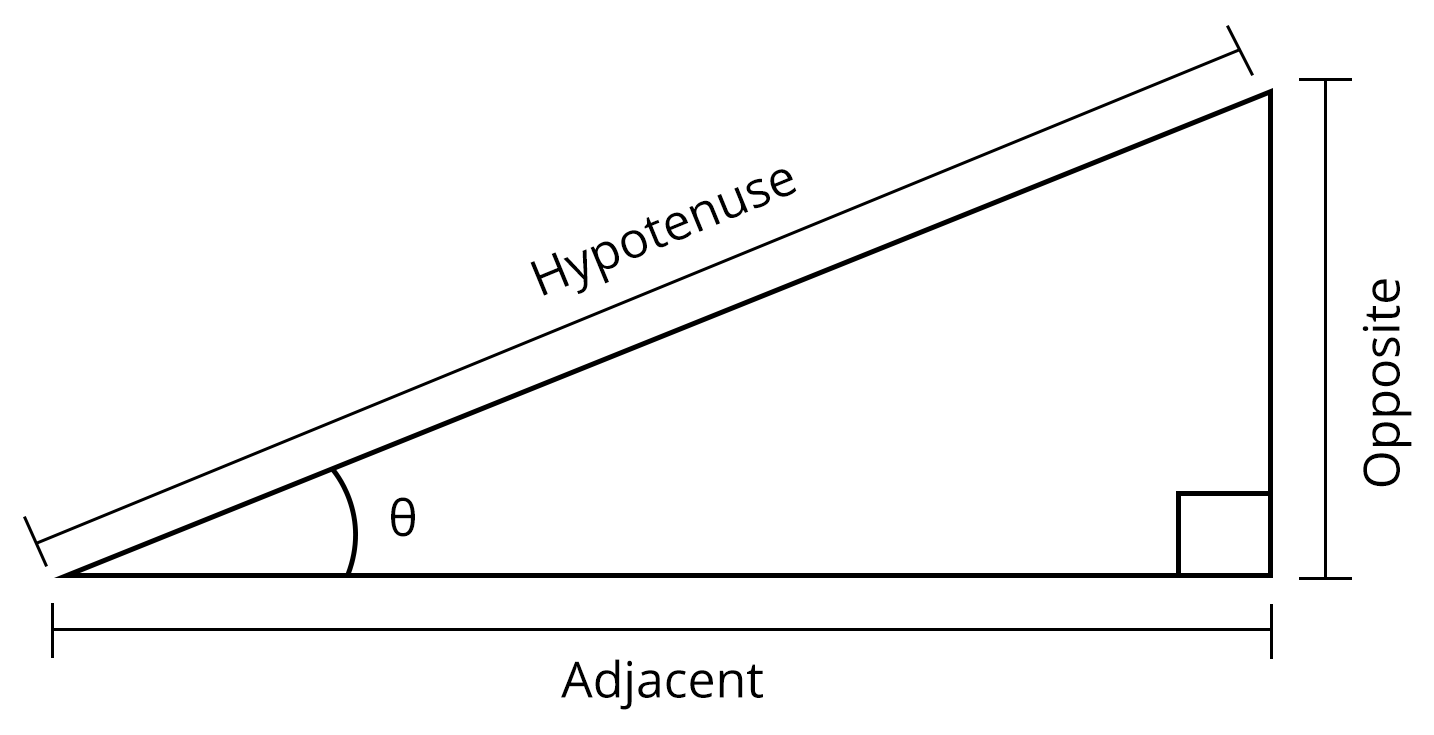Consider a right-angled triangle with the longest side being the hypotenuse and the sides opposite to the hypotenuse being the adjacent and opposite sides.

### Trigonometric Functions Formula

If $\theta$$\theta$ is the angle formed by the base and hypotenuse in a right-angled triangle, then

$\mathrm{sin}\theta =\frac{\text{Perpendicular}}{\text{Hypotenuse}}$$\sin \theta = \dfrac{\text{Perpendicular}}{\text{Hypotenuse}}$

$\mathrm{cos}\theta =\frac{\text{Base}}{\text{Hypotenuse}}$$\cos \theta = \dfrac{\text{Base}}{\text{Hypotenuse}}$

$\mathrm{tan}\theta =\frac{\text{Perpendicular}}{\text{Base}}$$\tan \theta = \dfrac{\text{Perpendicular}}{\text{Base}}$

The values of the other three functions, cot, sec, and cosec, are determined by the values of tan, cos, and sin.

$\mathrm{cot}\theta =\frac{1}{\mathrm{tan}\theta }=\frac{\text{Base}}{\text{Perpendicular}}$$\cot \theta = \dfrac{1}{\tan \theta} = \dfrac{\text{Base}}{\text{Perpendicular}}$

$\mathrm{sec}\theta =\frac{1}{\mathrm{cos}\theta }=\frac{\text{Hypotenuse}}{\text{Base}}$$\sec \theta = \dfrac{1}{\cos \theta} = \dfrac{\text{Hypotenuse}}{\text{Base}}$

$\text{cosec}\theta =\frac{1}{\mathrm{sin}\theta }=\frac{\text{Hypotenuse}}{\text{Perpendicular}}$$\text{cosec} \theta = \dfrac{1}{\sin \theta} = \dfrac{\text{Hypotenuse}}{\text{Perpendicular}}$

### Even and Odd Trigonometric Functions

Even or odd can be used to describe the trigonometric function.

Odd Trigonometric Functions: If f(-x) = -f(x) and symmetric with respect to the origin, a trigonometric function is said to be odd.

Even Trigonometric Functions: If f(-x) = f(x) and symmetric to the y-axis, a trigonometric function is said to be even.

• $\mathrm{sin}\left(-x\right)=-\mathrm{sin}x$$\sin (-x) = -\sin x$

• $\mathrm{cos}\left(-x\right)=\mathrm{cos}x$$\cos (-x) = \cos x$

• $\mathrm{tan}\left(-x\right)=-\mathrm{tan}x$$\tan (-x) = -\tan x$

• $\text{cosec}\left(-x\right)=-\text{cosec}x$$\text{cosec} (-x) = -\text{cosec} x$

• $\mathrm{sec}\left(-x\right)=\mathrm{sec}x$$\sec (-x) = \sec x$

• $\mathrm{cot}\left(-x\right)=-\mathrm{cot}x$$\cot (-x) = -\cot x$

### Trigonometric Functions in Different Quadrants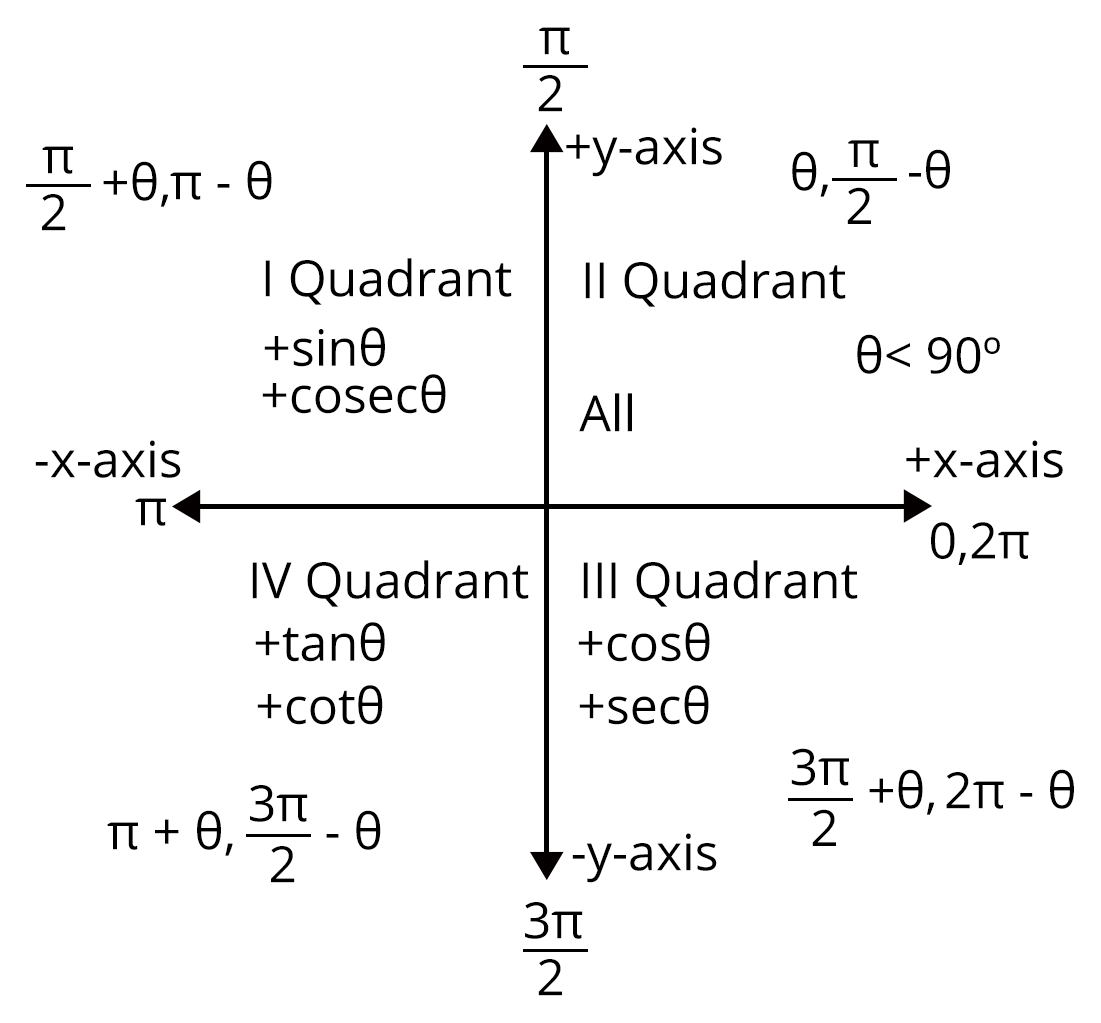I Quadrant II Quadrant $\mathrm{sin}\theta$$\sin \theta$ increases from 0 to 1 $\mathrm{sin}\theta$$\sin \theta$ decreases from 1 to 0 $\mathrm{cos}\theta$$\cos \theta$ decreases from 1 to 0 $\mathrm{cos}\theta$$\cos \theta$  decreases from 0 to -1 $\mathrm{tan}\theta$$\tan \theta$ increases from 0 to $\mathrm{\infty }$$\infty$ $\mathrm{tan}\theta$$\tan \theta$ increases from  $-\mathrm{\infty }$$-\infty$ to 0 $\mathrm{cot}\theta$$\cot \theta$ decreases from $\mathrm{\infty }$$\infty$ to 0 $\mathrm{cot}\theta$$\cot \theta$ decreases from 0 to $-\mathrm{\infty }$$-\infty$ $\mathrm{sec}\theta$$\sec \theta$ increases from 1 to $\mathrm{\infty }$$\infty$ $\mathrm{sec}\theta$$\sec \theta$ increases from $-\mathrm{\infty }$$-\infty$ to -1 $\text{cosec}\theta$$\text{cosec} \theta$ decreases from $\mathrm{\infty }$$\infty$ to 1 $\text{cosec}\theta$$\text{cosec} \theta$ decreases from 1 to $\mathrm{\infty }$$\infty$

 III Quadrant IV Quadrant $\mathrm{sin}\theta$$\sin \theta$ increases from 0 to -1 $\mathrm{sin}\theta$$\sin \theta$ increases from -1 to 0 $\mathrm{cos}\theta$$\cos \theta$ decreases from -1 to 0 $\mathrm{cos}\theta$$\cos \theta$  increases from 0 to 1 $\mathrm{tan}\theta$$\tan \theta$ increases from 0 to $\mathrm{\infty }$$\infty$ $\mathrm{tan}\theta$$\tan \theta$ increases from  $-\mathrm{\infty }$$-\infty$ to 0 $\mathrm{cot}\theta$$\cot \theta$ decreases from $\mathrm{\infty }$$\infty$ to 0 $\mathrm{cot}\theta$$\cot \theta$ decreases from 0 to $-\mathrm{\infty }$$-\infty$ $\mathrm{sec}\theta$$\sec \theta$ decreases from -1 to $-\mathrm{\infty }$$-\infty$ $\mathrm{sec}\theta$$\sec \theta$ decreases from $\mathrm{\infty }$$\infty$ to 1 $\text{cosec}\theta$$\text{cosec} \theta$ decreases from $-\mathrm{\infty }$$-\infty$ to -1 $\text{cosec}\theta$$\text{cosec} \theta$ decreases from -1 to $\mathrm{\infty }$$\infty$

### Trigonometric Table - Trigonometry Table Formula

The trigonometric table is made up of interrelated trigonometric ratios such as sine, cosine, tangent, cosecant, secant, and cotangent are used to calculate standard angle values. Refer to the below trigonometric table chart to know more about these ratios.

 Angles ${0}^{\circ }$$0^\circ$ ${30}^{\circ }$$30^\circ$ ${45}^{\circ }$$45^\circ$ ${60}^{\circ }$$60^\circ$ ${90}^{\circ }$$90^\circ$ $\mathrm{sin}\theta$$\sin \theta$ 0 $\frac{1}{2}$$\dfrac{1}{2}$ $\frac{1}{\sqrt{2}}$$\dfrac{1}{\sqrt 2}$ $\frac{\sqrt{3}}{2}$$\dfrac{\sqrt 3}{2}$ 1 $\mathrm{cos}\theta$$\cos \theta$ 1 $\frac{\sqrt{3}}{2}$$\dfrac{\sqrt 3}{2}$ $\frac{1}{\sqrt{2}}$$\dfrac{1}{\sqrt 2}$ $\frac{1}{2}$$\dfrac{1}{2}$ 0 $\mathrm{tan}\theta$$\tan \theta$ 0 $\frac{1}{\sqrt{3}}$$\dfrac{1}{\sqrt 3}$ 1 $\sqrt{3}$$\sqrt{3}$ $\mathrm{\infty }$$\infty$ $\mathrm{sec}\theta$$\sec \theta$ $\mathrm{\infty }$$\infty$ $2$$2$ $\sqrt{2}$${\sqrt 2}$ $\frac{2}{\sqrt{3}}$$\dfrac{2}{\sqrt 3}$ 1 $\text{cosec}\theta$$\text{cosec} \theta$ 1 $\frac{2}{\sqrt{3}}$$\dfrac{2}{\sqrt 3}$ $\sqrt{2}$${\sqrt 2}$ 2 $\mathrm{\infty }$$\infty$ $\mathrm{cot}\theta$$\cot \theta$ $\mathrm{\infty }$$\infty$ $\sqrt{3}$$\sqrt 3$ 1 $\frac{1}{\sqrt{3}}$$\dfrac{1}{\sqrt 3}$ 0

Similarly, we can find the trigonometric ratio values for angles other than 90 degrees, such as 180 degrees, 270 degrees, and 360 degrees.

### Important Trigonometric Angles

Trigonometric angles are the angles in a right-angled triangle that can be used to represent various trigonometric functions. ${0}^{\circ },{30}^{\circ },{45}^{\circ },{60}^{\circ }$$0^\circ, 30^\circ, 45^\circ, 60^\circ$ and ${90}^{\circ }$$90^\circ$ are some of the standard angles used in trigonometry. These angles' trigonometric values can be found directly in a trigonometric table. ${180}^{\circ },{270}^{\circ },$$180^\circ, 270^\circ,$ and ${360}^{\circ }$$360^\circ$ are some other important angles in trigonometry. The angle of trigonometry can be expressed in terms of trigonometric ratios as follows:

• $\theta ={\mathrm{sin}}^{-1}\left(\frac{\text{Perpendicular}}{\text{Hypotenuse}}\right)$$\theta = \sin^{-1} \left(\dfrac{\text{Perpendicular}}{\text{Hypotenuse}}\right)$

• $\theta ={\mathrm{cos}}^{-1}\left(\frac{\text{Base}}{\text{Hypotenuse}}\right)$$\theta = \cos^{-1} \left(\dfrac{\text{Base}}{\text{Hypotenuse}}\right)$

• $\theta ={\mathrm{tan}}^{-1}\left(\frac{\text{Perpendicular}}{\text{Base}}\right)$$\theta = \tan^{-1} \left(\dfrac{\text{Perpendicular}}{\text{Base}}\right)$

### Unit Circle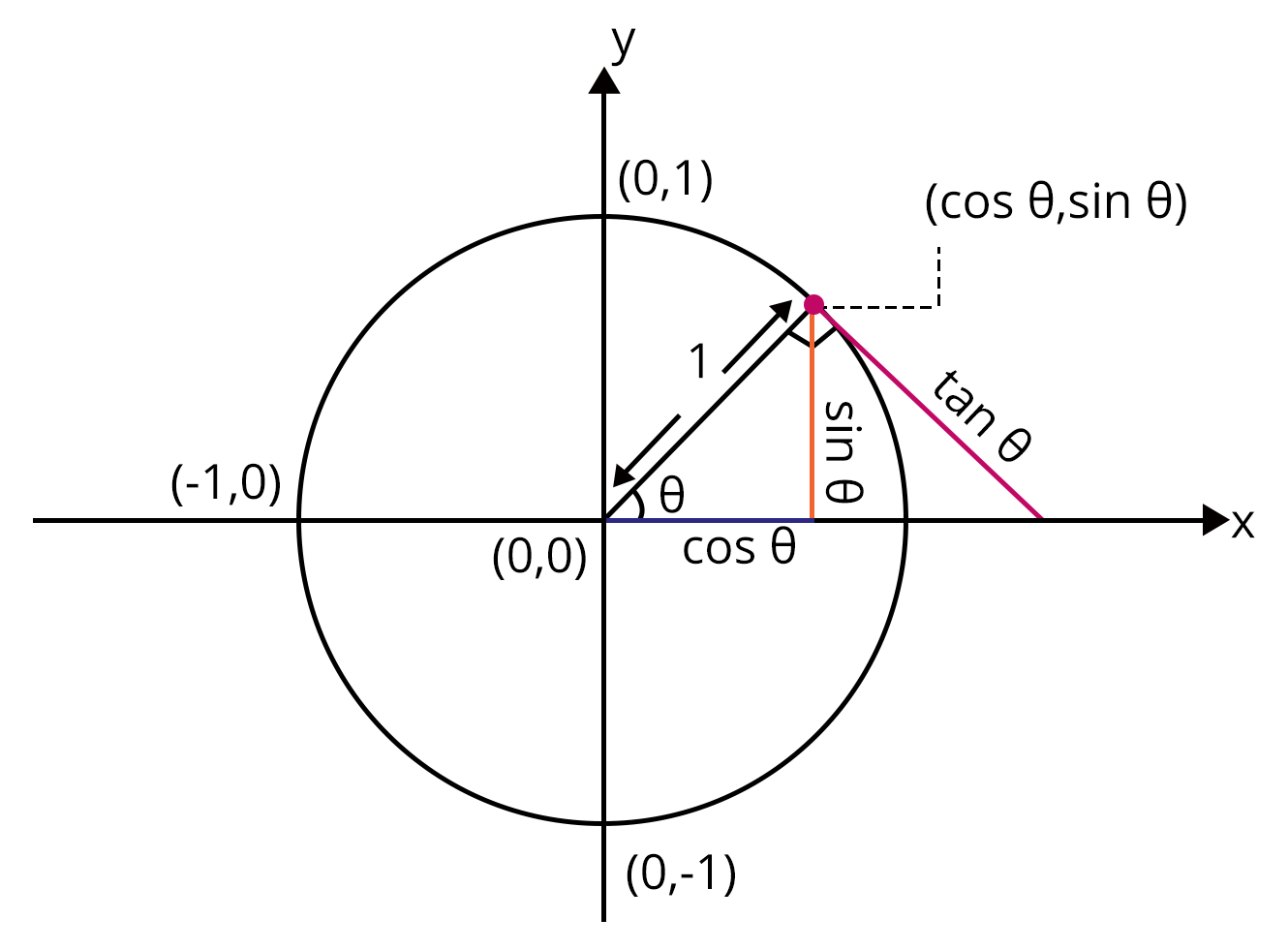Because the center of the circle is at the origin and the radius is 1, the concept of unit circle allows us to directly measure the angles of cos, sin, and tan. Assume theta is an angle, and the length of the perpendicular is y and the length of the base is x. The hypotenuse is the same length as the radius of the unit circle, which is 1. As a result, the trigonometry ratios can be written as;

$\mathrm{sin}\theta =y$$\sin \theta = y$

$\mathrm{cos}\theta =x$$\cos \theta = x$

$\mathrm{tan}\theta =\frac{y}{x}$$\tan \theta = \dfrac{y}{x}$

### List of Trigonometric Formulas

There are different formulas in trigonometry depicting the relationships between trigonometric ratios and the angles for different quadrants. The basic trigonometry formulas list is given below:

1. Pythagorean Identities

${\mathrm{sin}}^{2}\theta +{\mathrm{cos}}^{2}\theta =1$$\sin^{2} \theta+\cos^{2} \theta=1$

${\mathrm{tan}}^{2}\theta +1={\mathrm{sec}}^{2}\theta$$\tan^{2} \theta+1=\sec^{2} \theta$

${\mathrm{cot}}^{2}\theta +1={\text{cosec}}^{2}\theta$$\cot^{2} \theta+1=\text{cosec}^{2} \theta$

$\mathrm{sin}2\theta =2\mathrm{sin}\theta \mathrm{cos}\theta$$\sin 2 \theta=2 \sin \theta \cos \theta$

$\mathrm{cos}2\theta ={\mathrm{cos}}^{2}\theta -{\mathrm{sin}}^{2}\theta$$\cos 2 \theta=\cos^{2} \theta-\sin^{2} \theta$

$\mathrm{tan}2\theta =\frac{2\mathrm{tan}\theta }{1-{\mathrm{tan}}^{2}\theta }$$\tan 2 \theta=\dfrac{2 \tan \theta}{1-\tan^{2} \theta}$

$\mathrm{cot}2\theta =\frac{{\mathrm{cot}}^{2}\theta -1}{2\mathrm{cot}\theta }$$\cot 2 \theta=\dfrac{\cot^{2} \theta-1}{2 \cot \theta}$

2. Sine and Cosine Law in Trigonometry

Sine Law: $\frac{a}{\mathrm{sin}A}=\frac{b}{\mathrm{sin}B}=\frac{c}{\mathrm{sin}C}$$\dfrac{a}{\sin A} = \dfrac{b}{\sin B} = \dfrac{c}{\sin C}$

Cosine Law: ${c}^{2}={a}^{2}+{b}^{2}–2ab\mathrm{cos}C$$c^2 = a^2 + b^2 – 2ab \cos C$

${a}^{2}={b}^{2}+{c}^{2}–2bc\mathrm{cos}A$$a^2 = b^2 + c^2 – 2bc \cos A$

${b}^{2}={a}^{2}+{c}^{2}–2ac\mathrm{cos}B$$b^2 = a^2 + c^2 – 2ac \cos B$

The lengths of the triangle's sides are a, b, and c, and the triangle's angle is A, B, and C.

3. Sum and Difference identities

Let u and v be the angles:

$\mathrm{sin}\left(u+v\right)=\mathrm{sin}\left(u\right)\mathrm{cos}\left(v\right)+\mathrm{cos}\left(u\right)\mathrm{sin}\left(v\right)$$\sin ({u}+{v})=\sin ({u}) \cos ({v})+\cos ({u}) \sin ({v})$

$\mathrm{cos}\left(u+v\right)=\mathrm{cos}\left(u\right)\mathrm{cos}\left(v\right)-\mathrm{sin}\left(u\right)\mathrm{sin}\left(v\right)$$\cos ({u}+{v})=\cos ({u}) \cos ({v})-\sin ({u}) \sin ({v})$

$\mathrm{tan}\left(u+v\right)=\frac{\mathrm{tan}\left(u\right)+\mathrm{tan}\left(v\right)}{1-\mathrm{tan}\left(u\right)\mathrm{tan}\left(v\right)}$$\tan ({u}+{v})=\dfrac{\tan (u)+\tan (v)}{1-\tan (u) \tan (v)}$

$\mathrm{sin}\left(u-v\right)=\mathrm{sin}\left(u\right)\mathrm{cos}\left(v\right)-\mathrm{cos}\left(u\right)\mathrm{sin}\left(v\right)$$\sin ({u}-{v})=\sin ({u}) \cos ({v})-\cos ({u}) \sin ({v})$

$\mathrm{cos}\left(u-v\right)=\mathrm{cos}\left(u\right)\mathrm{cos}\left(v\right)+\mathrm{sin}\left(u\right)\mathrm{sin}\left(v\right)$$\cos ({u}-{v})=\cos ({u}) \cos ({v})+\sin ({u}) \sin ({v})$

$\mathrm{tan}\left(u-v\right)=\frac{\mathrm{tan}\left(u\right)-\mathrm{tan}\left(v\right)}{1+\mathrm{tan}\left(u\right)\mathrm{tan}\left(v\right)}$$\tan ({u}-{v})=\dfrac{\tan (u)-\tan (v)}{1+\tan (u) \tan (v)}$

4. Trigonometry Identities

${\mathrm{sin}}^{2}\theta +{\mathrm{cos}}^{2}\theta =1$$\sin^{2} \theta+\cos^{2} \theta=1$

${\mathrm{tan}}^{2}\theta +1={\mathrm{sec}}^{2}\theta$$\tan^{2} \theta+1=\sec^{2} \theta$

${\mathrm{cot}}^{2}\theta +1={\text{cosec}}^{2}\theta$$\cot^{2} \theta+1=\text{cosec}^{2} \theta$

5. Euler's Formula for trigonometry

${e}^{ix}=\mathrm{cos}x+i\mathrm{sin}x$$e^{i x}=\cos x+i \sin x$

Where $x$${x}$ is the angle and $i$${i}$ is the imaginary number.

Hence Euler’s formula for $\mathrm{sin},\mathrm{cos}$$\sin, \cos$ and $\mathrm{tan}$$\tan$ is:

$\mathrm{sin}x=\frac{{e}^{ix}-{e}^{-ix}}{2i}$$\sin x=\dfrac{e^{i x}-e^{-i x}}{2 i}$

$\mathrm{cos}x=\frac{{e}^{ix}+{e}^{-ix}}{2}$$\cos x=\dfrac{e^{i x}+e^{-i x}}{2}$

$\mathrm{tan}x=\frac{\left({e}^{ix}-{e}^{-ix}\right)}{i\left({e}^{ix}+{e}^{-ix}\right)}$$\tan x=\dfrac{\left(e^{i x}-e^{-i x}\right)}{i\left(e^{i x}+e^{-i x}\right)}$

### Trigonometry notes on Trigonometry Identities

• The trigonometry identities are the trigonometry equations that include all of the trigonometry ratios of all the angles

• Each trigonometric ratio can be expressed in terms of another trigonometric ratio.

• We can easily find the other value of the trigonometry ratio if we know one of the values of the trigonometry ratio.

• They can also be used to calculate trigonometric formulas.

### Application of Trigonometry

The height of a structure or a mountain is calculated using trigonometry. The height of a building can be easily calculated using trigonometric functions and the distance of a structure from the perspective. It is used in a variety of fields and has no specific applications in solving functional problems. For example, in the development of computer music, trigonometry is used: as you may know, sound travels in waves, and this wave pattern is used in the development of computer music by passing it through a sine or cosine function.

### Trigonometry Examples:

Example 1:  A man stands in front of a 44 foot pole. According to his calculations, the pole cast a shadow that was 13 feet long. Can you assist him in determining the sun's angle of elevation from the shadow's tip?

Ans: Let x be the angle of elevation of the sun,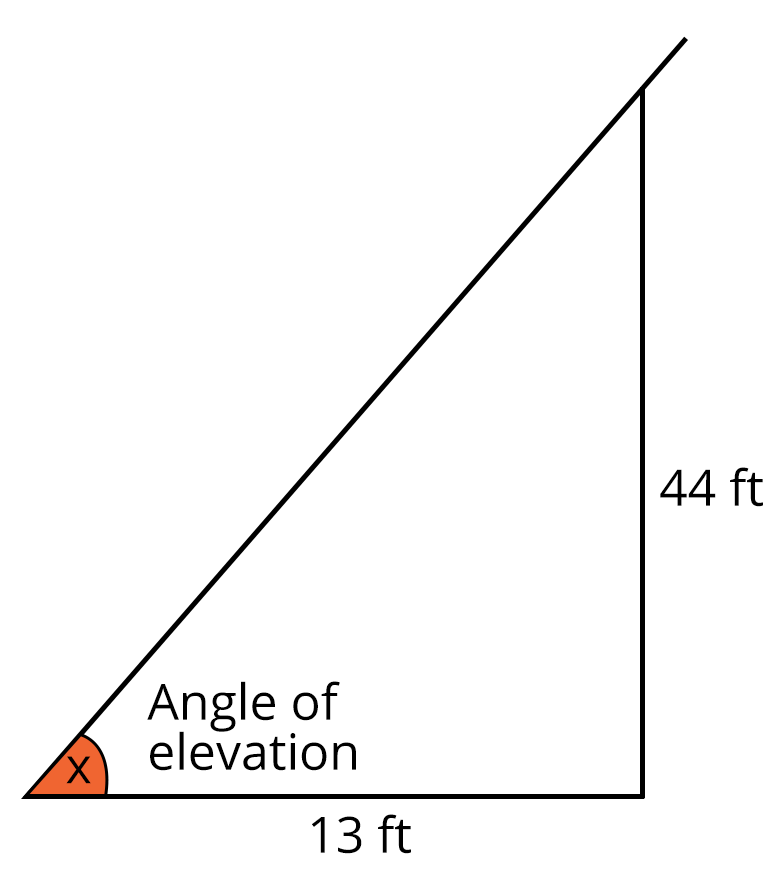$\mathrm{tan}x=\frac{44}{13}=3.384$$\tan x = \dfrac{44}{13} = 3.384$

$x={\mathrm{tan}}^{-1}\left(3.384\right)=1.283$$x = \tan^{-1}(3.384) = 1.283$

Hence, $x$$x$ in degree is ${73.54}^{\circ }$$73.54^\circ$

Example 2: Find the value of $\mathrm{sin}{75}^{\circ }$$\sin 75^\circ$

Ans: Given, $\mathrm{sin}{75}^{\circ }$$\sin 75^\circ$

To find the value of $\mathrm{sin}{75}^{\circ }$$\sin 75^\circ$ use the formula

$\mathrm{sin}\left(A+B\right)=\mathrm{sin}A\cdot \mathrm{cos}B+\mathrm{cos}A\cdot \mathrm{sin}B$$\sin(A + B) = \sin A \cdot \cos B + \cos A \cdot \sin B$

Split ${75}^{\circ }$$75^\circ$ such that $A={30}^{\circ }$$A = 30^\circ$ and $B={45}^{\circ }$$B = 45^\circ$

$\mathrm{sin}{75}^{\circ }=\mathrm{sin}\left({30}^{\circ }+{45}^{\circ }\right)$$\sin 75^\circ = \sin (30^\circ + 45^\circ)$

$⇒\mathrm{sin}{30}^{\circ }\cdot \mathrm{cos}{45}^{\circ }+\mathrm{cos}{30}^{\circ }\cdot \mathrm{sin}{45}^{\circ }$$\Rightarrow \sin30^\circ \cdot \cos 45^\circ + \cos 30^\circ \cdot \sin 45^\circ$

$⇒\frac{1}{2}\cdot \frac{1}{\sqrt{2}}+\frac{\sqrt{3}}{2}\cdot \frac{1}{\sqrt{2}}$$\Rightarrow \dfrac{1}{2} \cdot \dfrac{1}{\sqrt 2} + \dfrac{\sqrt 3}{2} \cdot \dfrac{1}{\sqrt 2}$

$⇒\frac{1}{2\sqrt{2}}+\frac{\sqrt{3}}{2\sqrt{2}}$$\Rightarrow \dfrac{1}{2\sqrt{2}} + \dfrac{\sqrt{3}}{2\sqrt{2}}$

$⇒\frac{\sqrt{3}+1}{2\sqrt{2}}$$\Rightarrow \dfrac{\sqrt{3} + 1}{2\sqrt{2}}$

### Solved problems of Previous Year Question

1. Find the general solution of $\mathrm{sin}x-3\mathrm{sin}2x+\mathrm{sin}3x=\mathrm{cos}x-3\mathrm{cos}2x+\mathrm{cos}3x$$\sin x - 3\sin 2x + \sin 3x = \cos x - 3\cos 2x + \cos 3x$ is _________.

Ans: $\mathrm{sin}x-3\mathrm{sin}2x+\mathrm{sin}3x=\mathrm{cos}x-3\mathrm{cos}2x+\mathrm{cos}3x$$\sin x - 3\sin 2x + \sin 3x = \cos x - 3\cos 2x + \cos 3x$

$⇒2\mathrm{sin}2x\mathrm{cos}x-3\mathrm{sin}2x-2\mathrm{cos}2x\mathrm{cos}x+3\mathrm{cos}2x=0$$\Rightarrow 2\sin 2x \cos x − 3 \sin 2x − 2 \cos 2x \cos x + 3 \cos 2x = 0$

$⇒\mathrm{sin}2x\left(2\mathrm{cos}x-3\right)-\mathrm{cos}2x\left(2\mathrm{cos}x-3\right)=0$$\Rightarrow \sin 2x (2\cos x − 3) − \cos 2x (2 \cos x − 3) = 0$

$⇒\left(\mathrm{sin}2x-\mathrm{cos}2x\right)\left(2\mathrm{cos}x-3\right)=0$$\Rightarrow (\sin 2x − \cos 2x) (2\cos x − 3) = 0$

$⇒\mathrm{sin}2x=\mathrm{cos}2x$$\Rightarrow \sin 2x = \cos 2x$

$⇒2x=2n\pi ±\left(\frac{\pi }{2}-2x\right)$$\Rightarrow 2x = 2n\pi \pm \left(\dfrac{\pi}{2} − 2x\right)$

$x=\frac{n\pi }{2}+\frac{\pi }{8}$$x = \dfrac{n\pi}{2} + \dfrac{\pi}{8}$

2. Find the value of $\mathrm{sin}\left({\mathrm{cot}}^{-1}x\right)$$\sin (\cot^{−1} x)$?

Ans: Let ${\mathrm{cot}}^{-1}x=\theta$$\cot^{−1} x = \theta$

Hence, $x=\mathrm{cot}\theta$$x = \cot \theta$

W.K.T $1+\mathrm{cot}2\theta =\text{cosec}2\theta$$1 + \cot 2\theta = \text{cosec} 2\theta$

$⇒1+{x}^{2}=\text{cosec}2\theta$$\Rightarrow 1 + x^2 = \text{cosec}2\theta$

W.K.T $\text{cosec }\theta = \dfrac{1}{\sin \theta}$

$⇒1+{x}^{2}=\frac{1}{{\mathrm{sin}}^{2}\theta }$$\Rightarrow 1+x^{2} =\dfrac{1}{\sin^{2} \theta}$

$⇒{\mathrm{sin}}^{2}\theta =\frac{1}{1+{x}^{2}}$$\Rightarrow \sin^{2} \theta =\dfrac{1}{1+x^{2}}$

$⇒\mathrm{sin}\theta =\frac{1}{\sqrt{1+{x}^{2}}}$$\Rightarrow \sin \theta = \dfrac{1}{\sqrt{1+x^{2}}}$

$\therefore \mathrm{sin}\left({\mathrm{cot}}^{-1}x\right)=\frac{1}{\sqrt{1+{x}^{2}}}$$\therefore \sin \left(\cot^{-1} x\right) = \dfrac{1}{\sqrt{1+x^{2}}}$

3. A balloon is observed simultaneously from three points A, B and C on a straight road directly under it. The angular elevation at B is twice and at C is thrice that of A. If the distance between A and B is 200 meters and the distance between B and C is 100 meters, then the height of the balloon is given by _________.

Ans: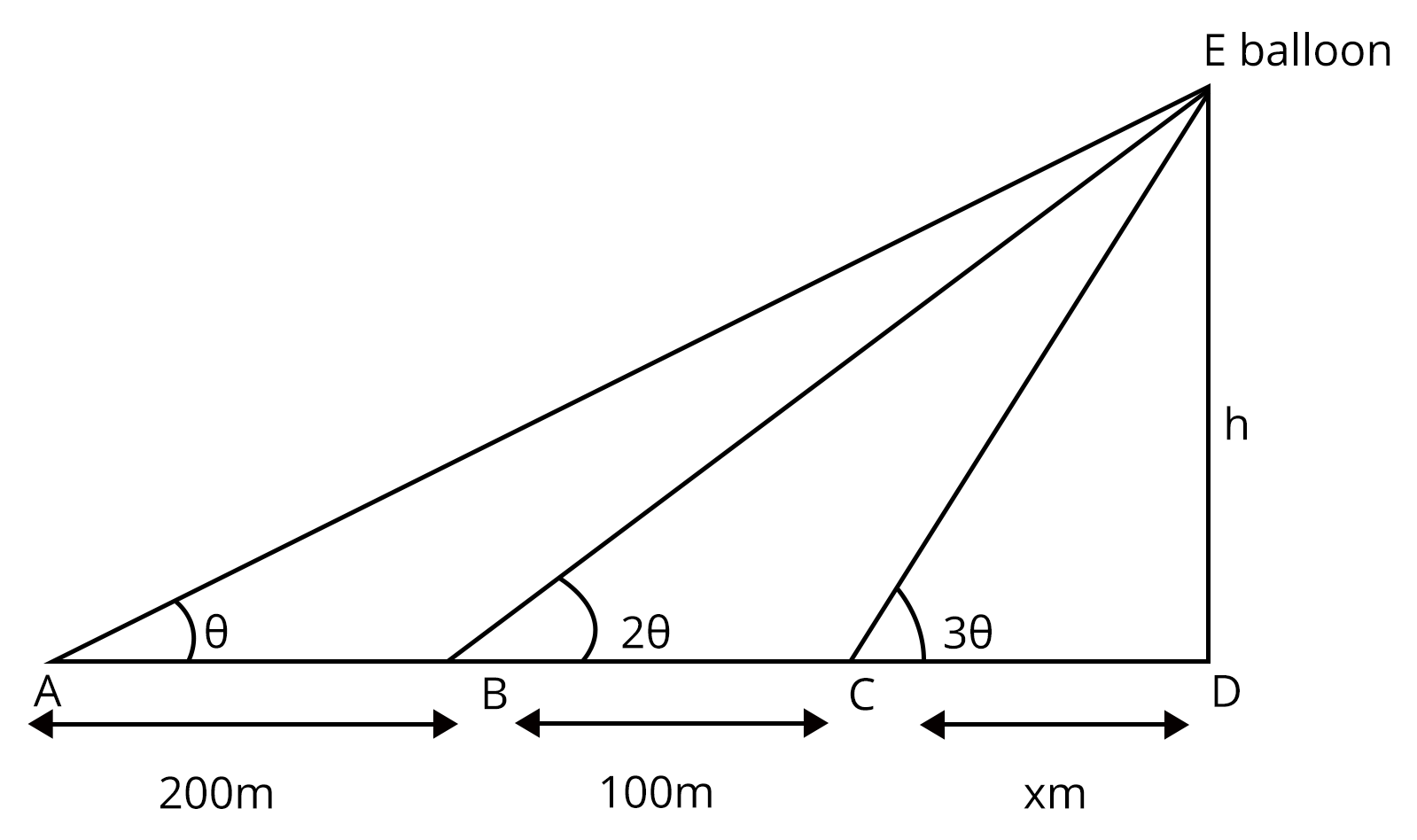$x=h\mathrm{cot}3\alpha$$x=h \cot 3 \alpha$ —(i)

$\left(x+100\right)=h\mathrm{cot}2\alpha$$(x+100)=h \cot 2 \alpha$ —(ii)

$x+300\right)=h\mathrm{cot}\alpha$${x}+300)={h} \cot \alpha$ —(iii)

From (i) and (ii), we get

$-100=h\left(\mathrm{cot}3\alpha -\mathrm{cot}2\alpha \right)=h\frac{\left(\mathrm{sin}2\alpha \mathrm{cos}3\alpha -\mathrm{cos}2\alpha \mathrm{sin}3\alpha \right)}{\mathrm{sin}3\alpha \mathrm{sin}2\alpha }=h\frac{\mathrm{sin}\left(3\alpha -2\alpha \right)}{\mathrm{sin}3\alpha \mathrm{sin}2\alpha }$$-100={h}(\cot 3 \alpha-\cot 2 \alpha)={h} \dfrac{(\sin 2 \alpha \cos 3 \alpha-\cos 2 \alpha \sin 3 \alpha)}{\sin 3 \alpha \sin 2 \alpha}=h\dfrac{\sin (3 \alpha-2 \alpha)}{\sin 3 \alpha \sin 2 \alpha}$

On simplifying we get,

$100=h\left(\frac{\mathrm{sin}\alpha }{\mathrm{sin}3\alpha \mathrm{sin}2\alpha }\right)$$100={h}\left(\dfrac{\sin \alpha}{\sin 3 \alpha \sin 2 \alpha}\right)$ —(iv)

Similarly,

From (ii) and (iii), we get

$-200=h\left(\mathrm{cot}2\alpha -\mathrm{cot}\alpha \right)=h\frac{\mathrm{sin}\left(2\alpha -\alpha \right)}{\mathrm{sin}2\alpha \mathrm{sin}\alpha }$$-200={h}(\cot 2 \alpha-\cot \alpha)=h\dfrac{\sin (2 \alpha-\alpha)}{\sin 2 \alpha \sin \alpha}$

On simplifying we get,

$200=h\left(\frac{\mathrm{sin}\alpha }{\mathrm{sin}2\alpha \mathrm{sin}\alpha }\right)$$200={h}\left(\dfrac{\sin \alpha}{\sin 2 \alpha \sin \alpha}\right)$ —(v)

Now divide equation (iv) and (v) we get,

$\frac{\mathrm{sin}3\alpha }{\mathrm{sin}\alpha }=\frac{200}{100}⇒\frac{\mathrm{sin}3\alpha }{\mathrm{sin}\alpha }=2$$\dfrac{\sin 3 \alpha}{\sin \alpha}=\dfrac{200}{100} \Rightarrow \dfrac{\sin 3 \alpha}{\sin \alpha}=2$ —(vi)

W.K.T $\mathrm{sin}3\alpha =3\mathrm{sin}\alpha -4{\mathrm{sin}}^{3}\alpha$$\sin 3 \alpha=3 \sin \alpha-4 \sin^{3} \alpha$

So, From equation (vi) we get,

$⇒3\mathrm{sin}\alpha -4{\mathrm{sin}}^{3}\alpha -2\mathrm{sin}\alpha =0$$\Rightarrow 3 \sin \alpha-4 \sin^{3} \alpha-2 \sin \alpha=0$

$⇒4{\mathrm{sin}}^{3}\alpha -\mathrm{sin}\alpha =0⇒\mathrm{sin}\alpha =0$$\Rightarrow 4 \sin^{3} \alpha-\sin \alpha=0 \Rightarrow \sin \alpha=0$ or ${\mathrm{sin}}^{2}\alpha =\frac{1}{4}$$\sin^{2} \alpha=\dfrac{1}{4}$

${\mathrm{sin}}^{2}\alpha =\frac{1}{4}={\mathrm{sin}}^{2}\left(\frac{\pi }{6}\right)$$\sin^{2} \alpha = \dfrac{1}{4} = \sin^{2}\left(\dfrac{\pi}{6}\right)$

$⇒\alpha =\frac{\pi }{6}$$\Rightarrow \alpha=\dfrac{\pi}{6}$

Hence

$h=200\mathrm{sin}2\alpha =200\mathrm{sin}\frac{\pi }{3}=200\frac{\sqrt{3}}{2}=100\sqrt{3}$${h}=200 \sin 2 \alpha=200 \sin \dfrac{\pi}{3}=200 \dfrac{\sqrt{3}}{2}=100 \sqrt{3}$

So the height of the balloon is $100\sqrt{3}$$100 \sqrt{3}$

### Practice problems

1. Find the value of ${\mathrm{sec}}^{2}\left({\mathrm{tan}}^{-1}2\right)+{\text{cosec}}^{2}\left({\mathrm{cot}}^{-1}3\right)=$$\sec^2 (\tan^{−1} 2) + \text{cosec}^2 (\cot^{−1} 3) =$ _________.

Ans: 15

2. If ${\mathrm{cos}}^{-1}p+{\mathrm{cos}}^{-1}q+{\mathrm{cos}}^{-1}r=\pi$$\cos^{−1} p + \cos^{−1} q + \cos^{−1} r = \pi$ then ${p}^{2}+{q}^{2}+{r}^{2}+2pqr=$$p^2 + q^2 + r^2 + 2pqr =$ ________.

Ans: 1

### Conclusion

Although trigonometry does not have many practical applications, it does make it easier to work with triangles. It's an excellent addition to geometry and actual measurements. With trigonometry, you can easily find the height without actually climbing a tree. They have a wide range of applications in real life and are extremely useful to most architects and astronomers. A standard trigonometry table helps solve subject-related problems. The 3 basic measures are sin, cos, and tan, and the remaining three are calculated using the formula given in the above list of formulas.

## Study Materials for Trigonometry:

These study materials will aid you in comprehending Trigonometry, ensuring a solid foundation for further mathematical pursuits.

 Trigonometry-Related Study Materials Trigonometry JEE Main Notes Trigonometry JEE Main Important Questions Trigonometry JEE Main Practice Paper

Access a curated collection of study materials and tips to excel in JEE Main and Advanced 2023-24, helping you prepare effectively for these prestigious engineering entrance exams.

Last updated date: 30th Sep 2023
Total views: 3.3k
Views today: 1.03k

## FAQs on JEE Important Chapter - Trigonometry

1. Who is the founder of trigonometry?

A Greek astronomer, geographer and mathematician, Hipparchus discovered the concept of trigonometry.

2. What Does $\theta$ Mean in Trigonometry?

In trigonometry, $\theta$ is used to represent a measured angle as a variable. It's the angle formed by the horizontal plane and the line of sight from the observer's eye to a higher object. Depending on the object's position, it's called the angle of elevation or the angle of depression. When the object is above the horizontal line, it's called the angle of elevation, and when it's below the horizontal line, it's called the angle of depression.

3. What is the best way to find trigonometric functions?

The ratio of the sides of a right-angled triangle is the trigonometric function. The Pythagorean rule Hypotenuse2 = Altitude2 + Base2 is also applied. In addition, the trigonometric functions have different values for different angles between the hypotenuse and the right triangle's base.